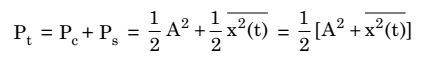# Power In AM Wave

## Power In AM Wave

You may note from the expression of AM wave that the carrier component of the amplitude modulated wave has the same amplitude as unmodulated carrier.And in addition to carrier component, the modulated wave consists of two sideband components.

It means that the modulated wave contains more power than the unmodulated carrier.

However, since the amplitudes of two sidebands depend upon the modulation index, it may be anticipated that the total power of the amplitude modulated wave would depend upon the modulation index also.

Here in this article, we will find the power contents of the carrier as well as the sidebands.

The general expression of AM wave is given as :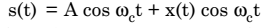The total power P of the AM wave is the sum of the carrier power (Pc)and sideband power (Ps).

### Carrier Power

The carrier power Pc  is equal to the mean-square (ms) value of the carrier term A cosωct i.e.,

Pc = mean square value of A cosωct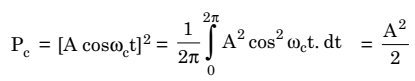### Sideband Power

The sideband power Ps is equal to the mean square value of the sideband term x(t) cosωct, i.e.,

Ps = mean square value of x(t) cosωct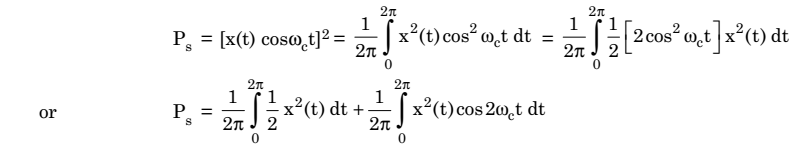In AM generation, a Band pass filter (BPF) or a tuned circuit tuned to carrier frequency ωc is used to filter out the second integral term. Therefore,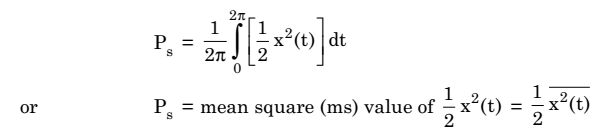However, the total sideband power Ps is due to the equal contributions of the upper and lower sidebands.

Hence, the power carried by the upper and the lower sidebands will be :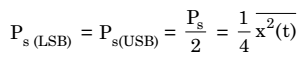Therefore, the total power Pt of the AM signal is the sum of the carrier power Pc and sideband power Ps.